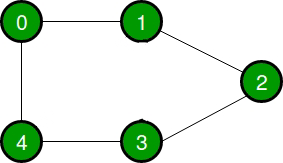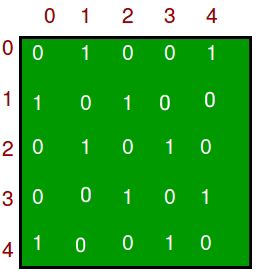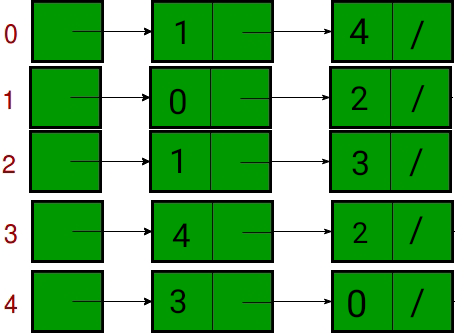A Graph is a non-linear data structure consisting of nodes and edges. The nodes are sometimes also referred to as vertices and the edges are lines or arcs that connect any two nodes in the graph. In this article, we will understand the difference between the ways of representation of the graph.

A graph can be represented in mainly two ways. They are:

1. Adjacency List: An Adjacency list is an array consisting of the address of all the linked lists. The first node of the linked list represents the vertex and the remaining lists connected to this node represents the vertices to which this node is connected. This representation can also be used to represent a weighted graph. The linked list can slightly be changed to even store the weight of the edge.
2. Adjacency Matrix: Adjacency Matrix is a 2D array of size V x V where V is the number of vertices in a graph. Let the 2D array be adj[][], a slot adj[i][j] = 1 indicates that there is an edge from vertex i to vertex j. Adjacency matrix for undirected graph is always symmetric. Adjacency Matrix is also used to represent weighted graphs. If adj[i][j] = w, then there is an edge from vertex i to vertex j with weight w.

Let us consider a graph to understand the adjacency list and adjacency matrix representation. Let the undirected graph be:The following graph is represented in the above representations as:

1. Adjacency Matrix: In the adjacency matrix representation, a graph is represented in the form of a two-dimensional array. The size of the array is V x V, where V is the set of vertices. The following image represents the adjacency matrix representation:2. Adjacency List: In the adjacency list representation, a graph is represented as an array of linked list. The index of the array represents a vertex and each element in its linked list represents the  vertices that form an edge with the vertex. The following image represents the adjacency list representation:The following table describes the difference between the adjacency matrix and the adjacency list:

table {
table-layout: fixed ;
width: 100% ;
}
td {
width: 25% ;
}

Attention reader! Don’t stop learning now. Get hold of all the important DSA concepts with the DSA Self Paced Course at a student-friendly price and become industry ready.

My Personal Notes arrow_drop_upCheck out this Author's contributed articles.

If you like GeeksforGeeks and would like to contribute, you can also write an article using contribute.geeksforgeeks.org or mail your article to contribute@geeksforgeeks.org. See your article appearing on the GeeksforGeeks main page and help other Geeks.

Please Improve this article if you find anything incorrect by clicking on the "Improve Article" button below.